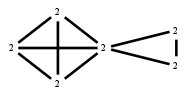# The Markov Bases Database

## G165g_bin

The binary graphical model of G165.

It is a hierarchical model of 6 variables. The dimension of the model is 21.The cardinality of the statespace is 64.

### Properties of the Markov basis

 Markov degree 2 336

 degree 2 336

The model has the following properties:

• All variables are binary.
• It is a graphical model.
• The minimal Markov basis is unique.
• The semigroup is normal.### FILES

 Markov basis: sufficient statistics matrix: G165g_bin.mar (63.33 kb) G165g_bin.mat (3.03 kb) G165g_bin.mod (35 b) G165g_bin.tar.gz (85.95 kb)